# Multiplying And Dividing Algebraic Fractions

Here we will learn about multiplying and dividing algebraic fractions, including algebraic fractions with monomial and binomial numerators and denominators.

There are also multiplying and dividing algebraic fractions worksheets based on Edexcel, AQA and OCR exam questions, along with further guidance on where to go next if you’re still stuck.

## What is multiplying and dividing algebraic fractions?

Multiplying and dividing algebraic fractions is the skill of multiplying and dividing two or more fractions that contain algebraic terms.

For example, \cfrac{2a}{5}, \cfrac{3-gh}{4f}, or \cfrac{1}{x-x^{2}} \, .

To do this we must combine our knowledge of multiplying and dividing fractions with our understanding of algebra.

Step-by-step guide: Multiplying and dividing fractions

Step-by-step guide: Algebraic terms

### What is multiplying and dividing algebraic fractions?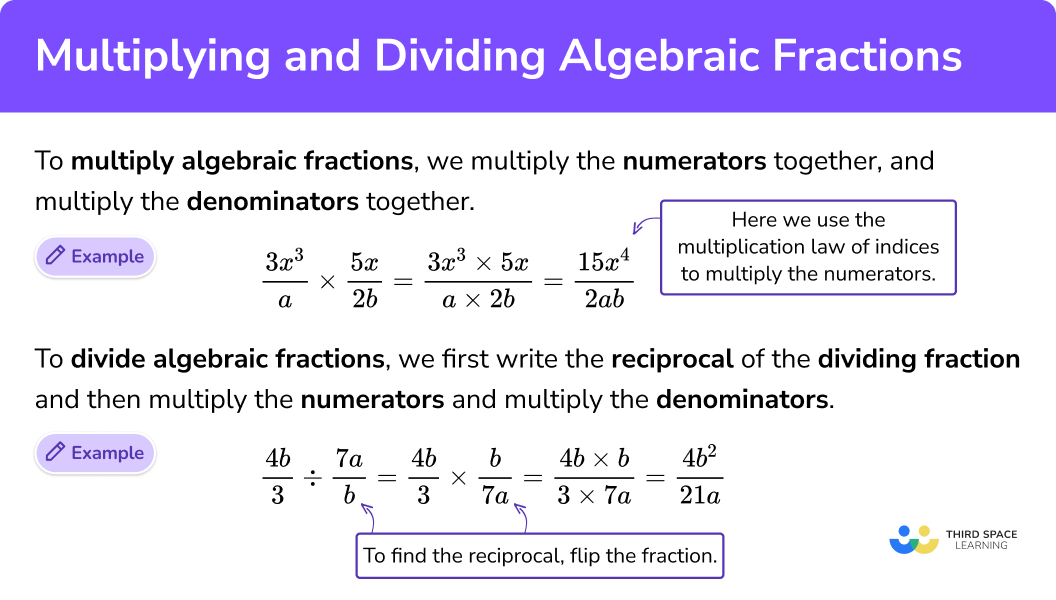### Multiplication with algebraic fractions

To multiply with fractions, we multiply the numerators together, and multiply the denominators together. This is the same for algebraic fractions, but we need to take extra care when multiplying algebraic terms or expressions.

For example, \cfrac{3x^3}{a} \times \cfrac{5x}{2b}=\cfrac{3x^3\times 5x}{a\times 2b}=\cfrac{15x^4}{2ab} \, .

### Division with algebraic fractions

To divide with fractions, we first write the reciprocal of the dividing fraction and then multiply the numerators together, and multiply the denominators together. This is the same for algebraic fractions, but we need to take extra care when multiplying algebraic terms or expressions.

For example, \cfrac{4b}{3} \div \cfrac{7a}{b}=\cfrac{4b}{3} \times \cfrac{b}{7a}=\cfrac{4b\times b}{3\times 7a}=\cfrac{4b^2}{21a} \, .

## How to multiply algebraic fractions

In order to multiply algebraic fractions:

1. Multiply the numerators together and multiply the denominators together.
2. Simplify the fraction if possible.

### Explain how to multiply algebraic fractions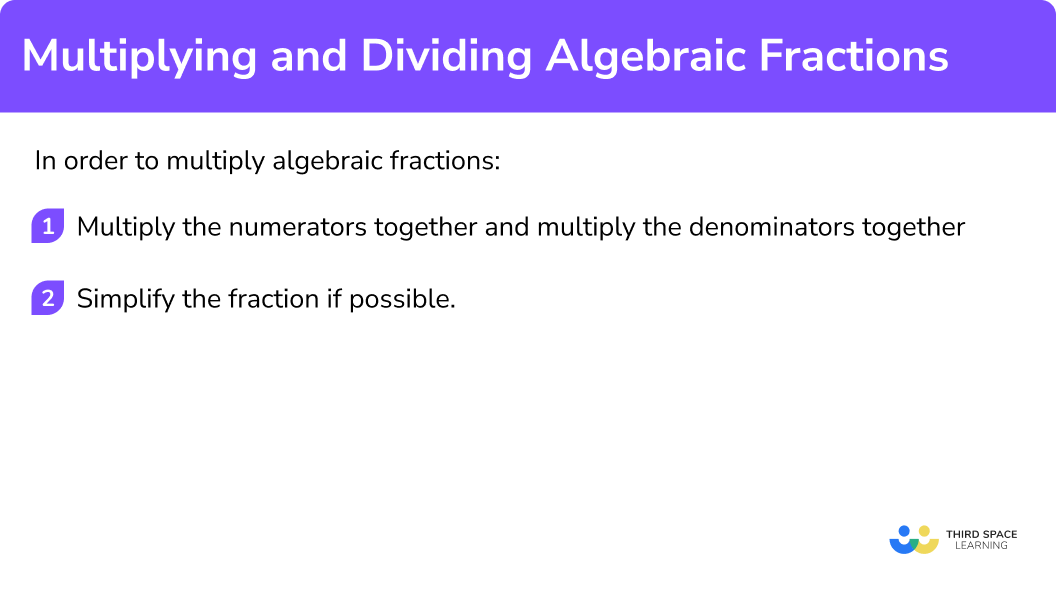## Multiplying algebraic fractions examples

### Example 1: multiplying algebraic fractions with monomial expressions.

Write as a single fraction in its simplest form, \cfrac{2}{a}\times\cfrac{5}{b} \, .

1. Multiply the numerators together and multiply the denominators together.

\cfrac{2\times5}{a\times b}=\cfrac{10}{ab}

2Simplify the fraction if possible.

The fraction \cfrac{10}{ab} cannot be simplified as the numerator and the denominator do not have any common factors.

### Example 2: multiplying algebraic fractions involving a binomial expression

Write as a single fraction in its simplest form, \cfrac{4x}{5}\times\cfrac{x+7}{8} \, .

\cfrac{4x\times(x+7)}{5\times8}=\cfrac{4x(x+7)}{40}

Note that you can leave algebraic products in factorised form. However, to help with step 2 it is best to check that they are fully factorised.

The terms in the bracket here do not share a common factor other than 1, and therefore the expression is fully factorised.

\cfrac{4x(x+7)}{40}

The numerator and the denominator have a common factor of 4 so we divide both the numerator and the denominator by 4 to simplify the fraction.

\cfrac{4x(x+7)\div4}{40\div4}=\cfrac{x(x+7)}{10}

If you expand the brackets then another way of writing the final answer is \cfrac{x^2+7x}{10}.

### Example 3: multiplying algebraic fractions involving binomial expressions

Write as a single fraction in its simplest form, \cfrac{3x+9}{4x}\times\cfrac{5}{2x+6} \, .

\cfrac{(3x+9)\times5}{4x\times(2x+6)}=\cfrac{5(3x+9)}{4x(2x+6)}

Note that you can leave algebraic products in factorised form. However, to help with step 2 it is best to check that they are fully factorised.

In this case the terms in the brackets of the numerator both have a factor of 3, and the terms in the brackets of the denominator both have a factor of 2. Therefore we can write the fraction like this.

\cfrac{5(3x+9)}{4x(2x+6)}=\cfrac{5\times3(x+3)}{4x\times2(x+3)}=\cfrac{15(x+3)}{8x(x+3)}

\cfrac{15(x+3)}{8x(x+3)}

We can simplify this fraction as the numerator and the denominator share the binomial factor (x+3).

\cfrac{15\cancel{(x+3)}}{8x\cancel{(x+3)}}=\cfrac{15}{8x}

## How to divide algebraic fractions

In order to divide algebraic fractions:

1. Find the reciprocal of the dividing fraction and rewrite the question with multiplication instead of division.
2. Multiply the numerators together and multiply the denominators together.
3. Simplify the fraction if possible.

### Expain how to divide algebraic fractions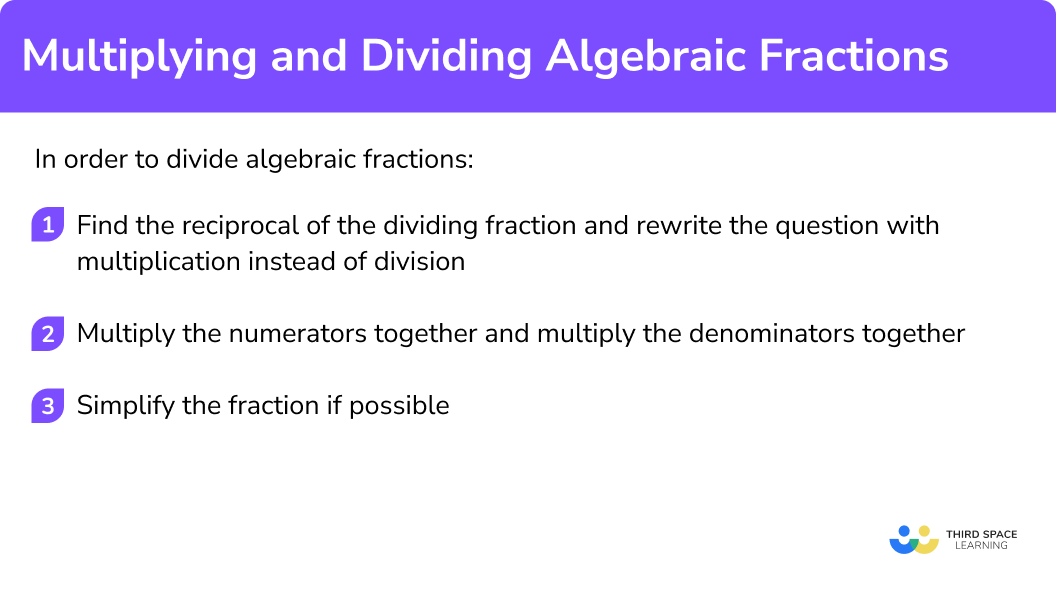## Dividing algebraic fractions examples

### Example 4: dividing with algebraic fractions with monomial expressions

Write as a single fraction in its simplest form, \cfrac{10}{3c}\div\cfrac{5}{3d} \, .

\cfrac{10}{3c}\div\cfrac{5}{3d}=\cfrac{10}{3c}\times\cfrac{3d}{5}

\cfrac{10}{3c}\times\cfrac{3d}{5}=\cfrac{10\times 3d}{3c\times5}=\cfrac{30d}{15c}

\cfrac{30d}{15c}

Both the numerator and the denominator have a common factor of 15 so we divide both the numerator and the denominator by 15 to simplify the fraction.

\cfrac{30d\div15}{15c\div15}=\cfrac{2d}{c}

### Example 5: dividing with algebraic fractions involving a binomial expression

Write as a single fraction in its simplest form, \cfrac{3x}{4}\div\cfrac{x+1}{8} \, .

\cfrac{3x}{4}\div\cfrac{x+1}{8}=\cfrac{3x}{4}\times\cfrac{8}{x+1}

\cfrac{3x}{4}\times\cfrac{8}{x+1}=\cfrac{3x\times 8}{4\times(x+1)}=\cfrac{24x}{4(x+1)}

Note that you can leave algebraic products in factorised form. However, to help with step 2 it is best to check that they are fully factorised.

The terms in the bracket here do not share a common factor other than 1, and therefore the expression is fully factorised.

\cfrac{24x}{4(x+1)}

Both the numerator and the denominator have a common factor of 4 so we divide both the numerator and the denominator by 4 to simplify the fraction.

\cfrac{24x}{4(x+1)}=\cfrac{24x\div4}{4(x+1)\div4}=\cfrac{6x}{x+1}

### Example 6: dividing with algebraic fractions with binomial expressions

Write as a single fraction in its simplest form, \cfrac{x^{2}-9}{x+1}\div\cfrac{x+3}{2} \, .

\cfrac{x^{2}-9}{x+1}\div\cfrac{x+3}{2}=\cfrac{x^{2}-9}{x+1}\times\cfrac{2}{x+3}

\cfrac{x^{2}-9}{x+1}\times\cfrac{2}{x+3}=\cfrac{(x^{2}-9)\times2}{(x+1)\times(x+3)}=\cfrac{2(x^{2}-9)}{(x+1)(x+3)}

Note that you can leave algebraic products in factorised form. However, to help with step 2 it is best to check that they are fully factorised.

The bracket of the numerator is the difference of two squares and can be factorised into double brackets.

\cfrac{2(x^{2}-9)}{(x+1)(x+3)}=\cfrac{2(x-3)(x+3)}{(x+1)(x+3)}

\cfrac{2(x+3)(x-3)}{(x+1)(x+3)}

We can now cancel a common binomial factor of (x+3).

\cfrac{2\cancel{(x+3)}(x-3)}{(x+1)\cancel{(x+3)}}=\cfrac{2(x-3)}{x+1}

Step-by-step guide: Difference of two squares

### Common misconceptions

• Cross multiplying vs multiplying fractions
When multiplying fractions, we multiply the numerators together, and multiply the denominators together. Some students can confuse this with a method referred to as ‘cross multiplying’ which some people teach as a method for dividing by a fraction.

For example,
Incorrectly choosing to cross multiply,

The correct solution is

• Common denominators are not needed
When we add or subtract fractions we must ensure there is a common denominator. A mistake often made by students is to believe this rule also applies to the multiplication of fractions. When we are multiplying fractions, we can choose to write the fractions with a common denominator but this simply makes the process lengthier and it is not necessary.

For example,
Without writing the fractions with a common denominator,

Writing the fractions with a common denominator first,

### Practice multiplying and dividing algebraic fractions questions

1) Write as a single fraction in the simplest form, \cfrac{3}{2e}\times\cfrac{8}{f}.

\cfrac{3f}{16e}\cfrac{16e}{3f}\cfrac{24}{2ef}\cfrac{12}{ef}\cfrac{3}{2e}\times\cfrac{8}{f}=\cfrac{3\times{8}}{2e\times{f}}=\cfrac{24}{2ef}=\cfrac{12}{ef}

2) Write as a single fraction in the simplest form, \cfrac{2x+3}{2}\times\cfrac{2(2x+1)}{3}.

\cfrac{6x+9}{4(2x+1)}\cfrac{4x^{2}+8x+3}{3}\cfrac{14x+13}{6}\cfrac{4x^{2}+8x+3}{6}\cfrac{2x+3}{2}\times\cfrac{2(2x+1)}{3}=\cfrac{(2x+3)\times{(2(2x+1))}}{6}=\cfrac{8x^{2}+16x+6}{6}=\cfrac{4x^{2}+8x+3}{3}

3) Write as a single fraction in the simplest form, \frac{4x+4}{7}\times\frac{3x}{2x+2}.

\cfrac{8(x+1)^{2}}{21x}\cfrac{12x^{2}+12}{14x+4}\cfrac{6x}{7}\cfrac{12x^{2}}{14}\cfrac{4x+4}{7}\times\cfrac{3x}{2x+2}=\cfrac{(4x+4)\times{3x}}{7\times(2x+2)}=\cfrac{3x\times{4(x+1)}}{7\times2(x+1)}=\cfrac{12x(x+1)}{14(x+1)}=\cfrac{6x}{7}

4) Write as a single fraction in the simplest form, \cfrac{15}{4x^{2}}\div\cfrac{3x}{2}.

\cfrac{5}{2x^{3}}\cfrac{13}{x}\cfrac{5}{2x}\cfrac{5}{2x^{2}}\cfrac{15}{4x^{2}}\div\cfrac{3x}{2}=\cfrac{15}{4x^{2}}\times\cfrac{2}{3x}=\cfrac{30}{12x^{3}}=\cfrac{5}{2x^{3}}

5) Write as a single fraction in the simplest form, \cfrac{5+x}{12}\div\cfrac{x^{2}}{4}.

\cfrac{1+x}{12-x^{2}}\cfrac{20+x}{12x^{2}}\cfrac{5+x}{3x^{2}}\cfrac{5}{3x}\cfrac{5+x}{12}\div\cfrac{x^{2}}{4}=\cfrac{5+x}{12}\times\cfrac{4}{x^{2}}=\cfrac{4(5+x)}{12x^{2}}=\cfrac{5+x}{3x^{2}}

6) Write as a single fraction in the simplest form, \cfrac{x^{2}-36}{4x-8}\div\cfrac{x-6}{2}.

\cfrac{x^{2}-72}{4(x-2)(x-6)}\cfrac{x-18}{2}\cfrac{x+6}{2(x-2)}\cfrac{6-x}{2x}\cfrac{x^{2}-36}{4x-8}\div\cfrac{x-6}{2}=\cfrac{x^{2}-36}{4x-8}\times\cfrac{2}{x-6}=\cfrac{(x^{2}-36)\times{2}}{(4x-8)\times(x-6)}=\cfrac{2(x+6)(x-6)}{4(x-2)(x-6)}

=\cfrac{2(x+6)}{4(x-2)}=\cfrac{x+6}{2(x-2)}

### Multiplying and dividing algebraic fractions GCSE questions

1. Write as a single fraction, \cfrac{2}{x}\times\cfrac{3}{y}\div\cfrac{5}{z}.

(3 marks)

\cfrac{6}{xy}\div\cfrac{5}{z}

(1)

\cfrac{6}{xy}\times\cfrac{z}{5}

(1)

\cfrac{6z}{5xy}

(1)

2. Triangle ABC is a right angled triangle where BC is perpendicular to AC and x > 0.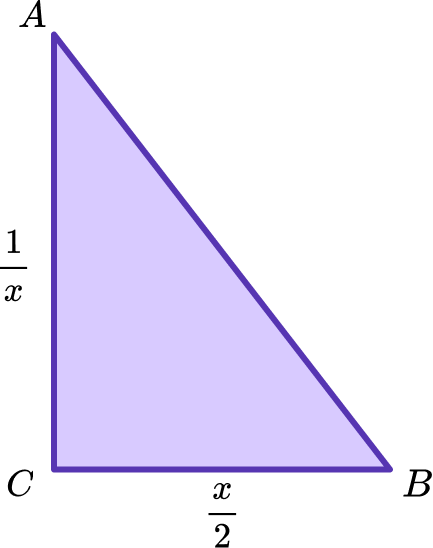(a) Calculate the area of the triangle.

(b) As the value of x increases, what happens to the area of the triangle?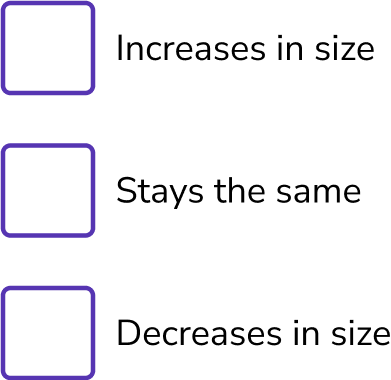(4 marks)

(a) (\cfrac{1}{x}\times\cfrac{x}{2})\div{2}

(1)

\cfrac{x}{2x}(=\cfrac{1}{2})\div{2}

\cfrac{1}{2}\times\cfrac{1}{2}

(1)

\cfrac{1}{4}

(1)

(b) Stays the same.

The area is independent of the value of x.

(1)

3. Use factorisation to fully simplify \cfrac{4x^{2}-16}{x+1}\div\cfrac{x-2}{3x+3} into the form a(x+b) where a and b are integers.

(5 marks)

4x^{2}-16=4(x^{2}-4)

(1)

4(x^{2}-4)=4(x+2)(x-2)

(1)

3x+3=3(x+1)

(1)

\cfrac{4(x+2)(x-2)}{x+1}\div\cfrac{x-2}{3(x+1)}=\cfrac{4(x+2)(x-2)}{x+1}\times\cfrac{3(x+1)}{x-2}

(1)

\cfrac{4(x+2)(x-2)\times{3}(x+1)}{(x+1)(x-2)}=12(x+2)

(1)

## Learning checklist

You have now learned how to:

• Multiply and divide with algebraic fractions

## Still stuck?

Prepare your KS4 students for maths GCSEs success with Third Space Learning. Weekly online one to one GCSE maths revision lessons delivered by expert maths tutors.

Find out more about our GCSE maths tuition programme.